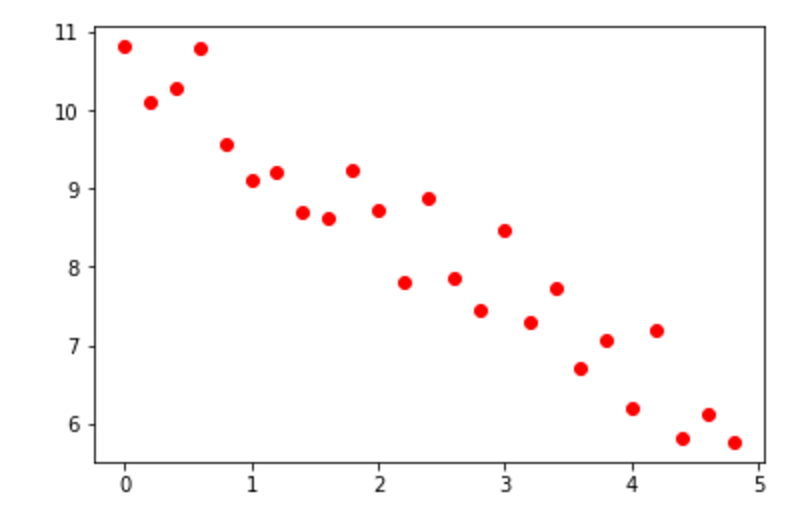### Machine Learning

If you’ve ever taken a class in statistics before, linear regression is probably a familiar concept. This is no coincidence. At its core, machine learning is about taking in information and expanding on it, so it's natural that techniques from statistics play an important role in machine learning.

It is possible to use statistical techniques to find a best-fit line, by first calculating five values about our data. If we represent our data sets as collections of points on a scatter plot, these values are the means of $x$ and $y$, the standard deviations of $x$ and $y$, and the correlation coefficient.

# Statistics of Linear Regression

If there are $n$ data points, then the mean of $x$ is simply the sum of all $x$ values divided by $n$. Correspondingly, the mean of $y$ is the sum of all $y$ values divided by $n$.

After calculating the means $($typically denoted as $\overline{x}$ and $\overline{y}),$ we can find the (sample) standard deviations for the data set through the following formulae: \begin{aligned} SD_x &= \sqrt{\frac{1}{(n-1)} \cdot \sum_{i=1}^{n} (x_i - \overline{x})^2} \\\\ SD_y &= \sqrt{\frac{1}{(n-1)} \cdot \sum_{i=1}^{n} (y_i - \overline{y})^2}. \end{aligned} The standard deviation of a data set gives a good idea of how close an average data point will be to the mean. A low standard deviation means that data points tend to cluster around the mean, while a large standard deviation usually means that they will be more spread out.

Out of the following sets of numbers, which will have the highest standard deviation? Use your intuition!

# Statistics of Linear Regression

Say that we are studying the correlation between voltage and a light bulb's brightness. Amazingly, we always know exactly what voltage we are using, but we haven’t been so lucky when measuring bulb brightness. Here are our options:

1. A perfectly accurate sensor taped to another light bulb, twice as bright as the one we’re measuring. We can’t get rid of the second light bulb; we don’t know why but the sensor won’t work without it.
2. A primitive but effective device from the 1840’s, that looks like a thermometer. It works, but a human must estimate its readings.
3. A completely broken machine. It always just reads zero.
4. Actual state-of-the-art technology, no quirks attached.

If we always run the light bulb with the same voltage and measure the brightness ten times, which of these devices will collect data with the highest standard deviation, all else being equal? Assume that the lightbulb is perfectly consistent and not a source of any fluctuations.

# Statistics of Linear Regression

After we have $SD_x$ and $SD_y,$ we only have the correlation coefficient, usually denoted by $r,$ left to calculate. This is difficult to calculate by hand, but the process for doing so is actually quite simple.

The first step is to convert $x$ and $y$ to standard units. For $1 \leq i \leq n,$ we must put each value $x_i$ through the formula $\frac{x_i-\overline{x}}{SD_x},$ which outputs the number of standard deviations $x_i$ is above the mean. We will call the updated value for $x_i$, $x_i^*$. Each value $y_i$ should be put through the analogous process with $\overline{y}$ and $SD_y.$

Now that we’ve changed our units, we can find our correlation coefficient by taking the average of the product of the updated $x$ and $y$ for each of our points.

This is given by $r = \frac{1}{n} \cdot \sum_{i=1}^{n} (x_i^*\cdot y_i^*).$ The formula for the correlation coefficient may seem inscrutable, but it’s actually quite easy to interpret. Its value ranges from $-1$ to $1,$ and it indicates how linearly correlated the data is.

If $r$ is close to zero, then the data is barely correlated at all, at least not with a linear relationship. However, if $r$ is close to $1,$ then the data is correlated and can be approximated well by a best-fit line with a positive slope. Conversely, if $r$ is close to $-1,$ the data is correlated and can be approximated well by a best-fit line with a negative slope.

# Statistics of Linear Regression

Which of the following values would be closest to the correlation coefficient of the graph below?# Statistics of Linear Regression

Now, with all this information, we can finally calculate a best-fit line. In standard units, this is simply the line $y^* = rx^*,$ where $r$ is the correlation coefficient. A little algebra will show that in the original units, this translates to the equation $y - \overline{y} = \frac{rSD_y}{SD_x}(x-\overline{x}).$ In other words, this version of the best-fit line has a slope of $\frac{rSD_y}{SD_x}$ and must pass through the point $(\overline{x}, \overline{y})$.

Although this line cannot go through every point in the data set, it does do a good job of representing them as a whole. It usually gives a good estimate for the expected value of $y$ at a given value of $x,$ at least if the relationship between the two is somewhat linear.

Alfred has worked hard analyzing his data, but he needs help with the last step. He has calculated that $\overline{x} = 50,$ $\overline{y} = 30,$ $SD_x=8,$ $SD_y=16,$ and $r=0.75.$ Which of the following equations gives the best-fit line he needs?

×